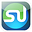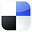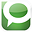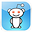# Using the Normal Distribution in Excelto Find Your Sales Ranges

This article will show you exactly how to use the Normal Distribution in Excel to calculate the probability that your daily sales are within one of two given ranges. The advantages of statistics in Excel to solve business statistics problems is that most problems can be solved in just one or two steps and there is no more need to look anything up on Normal Distribution tables.

As with any statistical test that uses or depends on the Normal Distribution, as assumption is made that your sales data is Normally Distributed. If you have at least 30 days of sample data, this is probably a reasonable assumption. Even so, you should always test for Normality prior to performing any parametric test or analysis.

Parametric means using or depending on a statistical distribution such as the Normal distribution. This blog contains a number of articles about Normality tests and nonparametric tests that are used in the event that your data is not Normally distributed.

In this problem, you are provided with the mean and standard deviation of your daily sales data and are then required to find the probability your sales from any random day will fall into one of two sales ranges. Here is the problem:

Problem: Use the Normal Distribution to Determine the Probability of Daily Sales Being in 1 of 2 Ranges

The daily unit sales of a commercial web site are normally distributed with a mean of 20 units sold daily and a standard deviation of 1 unit per day. Normality tests were performed on the sales data to verify that they are normally distributed.

The sales results from one random day are selected. What is the probability the sales from that day will be either more than 21.50 units sold OR less than 18.5 units sold?

Since we are solving for a probability (area under the Normal curve) for a Normal curve that is not standardized, use NORMDIST.

Probability of daily sales less than 18.5 units OR more than 21.5 units =

Probability of daily sales less than 18.5 PLUS Probability of daily sales greater than 21.5

The OR statement requires that the answer contains all elements of both sets.

The probability of less than 18.5 units sold in a single day

= NORMDIST(X, Sample Mean, Sample Standard Deviation, Cumulative?)

(Cumulative ask if we are calculating the
CDF - Cumulative Distribution Function - YES)

(Note that NORMDIST calculate the area LEFT of X)

=
NORMDIST(18.5,20,1,TRUE)

= 0.066807

= 6.67%

Click On Image To See Larger Version

OR (Plus)

The probability of more than 21.5 units sold in a single day
= 1 - NORMDIST(X, Sample Mean, Sample Standard Deviation, Cumulative?)

(Note that 1 - NORMDIST calculate area RIGHT of X)

=
1 - NORMDIST(21.5,20,1,TRUE)

= 1 - 0.933193 = 0.066807

= 6.67%
Click On Image To See Larger Version

The probability that daily daily are less than 18.5 OR greater than 21.5 = Probability of one day’s sales less than units + Probability of one day’s sales more than 21.5 units

= NORMDIST(18.5,20,1,TRUE) + [ 1 - NORMDIST(21.5,20,1,TRUE)

= 0.066807 + 0.066807

= 0.133614

= 13.36%

So the probability that a day’s sales are less than 18.5 units or more than 21.5 units = 13.36%

Click on Image To See Larger Version

Basic Description of Normal Distribution
The shape of the Normal distribution resembles a bell so it is sometimes called the "bell curve." The Normal curve is symmetric about its mean in the middle, and its tails on either side extend to infinity. The Normal distribution is a continuous function, not a discrete function, This means that any value can be graphed somewhere on a Normal curve. Discrete functions only map specific values, such as whole numbers.

Mapping the Normal Distribution
Any Normal distribution can be completely mapped if only the following two parameters are known:

1) Mean - µ - the Greek symbol "mu"

2) Standard deviation - σ - the Greek symbol "sigma"

If the mean and standard deviation of a Normal distribution are known, then every point of the Normal curve can be mapped.

*************************************************************

A Standardized Normal Curve is a Normal curve that has mean = 0 and standard deviation = 1

*************************************************************

The "68 - 95 - 99.7%" Rule
for the Normal distribution states that:

68% of all observations lie within 1 standard deviation of the mean, within the range of µ +/- σ

95% of all observations lie within 2 standard deviations of the mean, within the range of µ +/- 2σ

99.7% of all observations lie within 3 standard deviations of the mean, within the range of µ +/- 3σ

Click Image To See Larger Version

Click Image To See Larger Version

*****************************************

Using Normal Distribution in Excel To Find Your Sales Ranges

If You Like This, Then Share It...<

Excel Master Series Blog Directory

Statistical Topics and Articles In Each Topic

• Histograms in Excel
• Bar Chart in Excel
• Combinations & Permutations in Excel
• Normal Distribution in Excel
• t-Distribution in Excel
• Binomial Distribution in Excel
• z-Tests in Excel
• t-Tests in Excel
• Hypothesis Tests of Proportion in Excel
• Chi-Square Independence Tests in Excel
• Chi-Square Goodness-Of-Fit Tests in Excel
• F Tests in Excel
• Correlation in Excel
• Pearson Correlation in Excel
• Spearman Correlation in Excel
• Confidence Intervals in Excel
• Simple Linear Regression in Excel
• Multiple Linear Regression in Excel
• Logistic Regression in Excel
• Single-Factor ANOVA in Excel
• Two-Factor ANOVA With Replication in Excel
• Two-Factor ANOVA Without Replication in Excel
• Creating Interactive Graphs of Statistical Distributions in Excel
• Solving Problems With Other Distributions in Excel
• Optimization With Excel Solver
• Chi-Square Population Variance Test in Excel
• Analyzing Data With Pivot Tables
• SEO Functions in Excel
• Time Series Analysis in Excel

1.2.